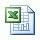# Amount of cuttings produced per foot of hole and total solid generated

After learning about capacity calculation, we can apply the capacity calculation to determine how much barrels of cutting produced per foot of hole drilled and total solid generated in pounds.

Use formula#1 and #2 for calculating amount of cutting generated per feet drilled.

## Formula#1 for BARRELS of cuttings drilled per foot of hole drilled:

Barrels of cutting per foot drilled = Dh2 x (1 – % porosity) ÷1029.4

Where: Dh is hole diameter in inch.

Example: Determine barrels of cuttings drilled for one foot of 6-1/8 inch hole with 25% (0.25) porosity:

Barrels/footage drilled = 6.1252 x (1 – 0.25) ÷1029.4
Barrels/footage drilled = 0.02733 bbl/footage drilled

## Formula#2 for CUBIC FEET of cuttings drilled per foot of hole drilled:

Cubic feet of cutting per foot drilled = Dh2 x 0.7854 x (1 – % porosity) ÷144

Where: Dh is hole diameter in inch.

Example: Determine barrels of cuttings drilled for one foot of 6-1/8 inch hole with 25% (0.25) porosity

Cubic feet/footage drilled = 6.1252 x 0.7854 x (1 – 0.25) ÷144
Cubic feet/footage drilled = 0.153462 cu ft/footage drilled

Moreover, you also apply sample density and volume relationship to determine total solids generated. Use the following formula to calculate total solid generated.

Wcg = 350 x Ch x L x (l – porosity) x Cutting density

Where;

Wcg = solids generated in pounds
Ch = capacity of hole in bbl/ft
L = footage drilled in ft
Cutting density = cutting density in gm/cc

Example: Determine the total pounds of solids generated in drilling 100 ft of  6-1/8 inch hole (0.03644 bbl/ft).

Density of cuttings = 2.20 gm/cc.

Porosity = 25%:
Wcg = 350 x 0.03644 x 100 x (1 – 0.25) x 2.2
Wcg = 2104.41 poundsPlease find the excel sheet how to calculate how much cuttings drilled per foot of hole drilled and total solids generated

Ref books: Lapeyrouse, N.J., 2002. Formulas and calculations for drilling, production and workover, Boston: Gulf Professional publishing.

Bourgoyne, A.J.T., Chenevert , M.E. & Millheim, K.K., 1986. SPE Textbook Series, Volume 2: Applied Drilling Engineering, Society of Petroleum Engineers.

Mitchell, R.F., Miska, S. & Aadny, B.S., 2011. Fundamentals of drilling engineering, Richardson, TX: Society of Petroleum Engineers.

Share the joy